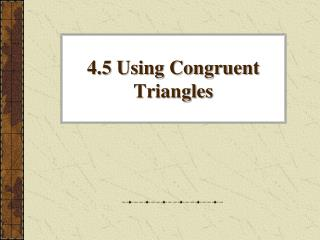Download Presentation4.5 Using Congruent Triangles

# 4.5 Using Congruent Triangles

Download Presentation## 4.5 Using Congruent Triangles

- - - - - - - - - - - - - - - - - - - - - - - - - - - E N D - - - - - - - - - - - - - - - - - - - - - - - - - - -
##### Presentation Transcript

1. 4.5 Using Congruent Triangles

2. Objectives/Assignment • Use congruent triangles to plan and write proofs • Use congruent triangles to prove constructions are valid • Assignment: 2-18 even, 26-36 even

3. Goal 1: Planning a Proof • Knowing that all pairs of corresponding parts of congruent triangles are congruent can help you reach conclusions about congruent figures.

4. For example, suppose you want to prove that PQS ≅ RQS in the diagram shown at the right. One way to do this is to show that ∆PQS ≅ ∆RQS by the SSS Congruence Postulate. Then you can use the fact that corresponding parts of congruent triangles are congruent to conclude that PQS ≅ RQS. Planning a Proof

5. Given: AB ║ CD, BC ║ AD Prove: AB≅CD Plan for proof: Show that ∆ABD ≅ ∆CDB. Then use the fact that corresponding parts of congruent triangles are congruent. Example 1: Planning & Writing a Proof

6. Solution: First copy the diagram and mark it with the given information. Then mark any additional information you can deduce. Because AB and CD are parallel segments intersected by a transversal, and BC and DA are parallel segments intersected by a transversal, you can deduce that two pairs of alternate interior angles are congruent. Example 1: Planning & Writing a Proof

7. Because AD ║CD, it follows from the Alternate Interior Angles Theorem that ABD ≅CDB. For the same reason, ADB ≅CBD because BC║DA. By the Reflexive property of Congruence, BD ≅ BD. You can use the ASA Congruence Postulate to conclude that ∆ABD ≅ ∆CDB. Finally because corresponding parts of congruent triangles are congruent, it follows that AB ≅ CD. Example 1: Paragraph Proof

8. Given: A is the midpoint of MT, A is the midpoint of SR. Prove: MS ║TR. Plan for proof: Prove that ∆MAS ≅ ∆TAR. Then use the fact that corresponding parts of congruent triangles are congruent to show that M ≅ T. Because these angles are formed by two segments intersected by a transversal, you can conclude that MS ║ TR. Example 2: Planning & Writing a Proof

9. Statements: A is the midpoint of MT, A is the midpoint of SR. MA ≅ TA, SA ≅ RA MAS ≅ TAR ∆MAS ≅ ∆TAR M ≅ T MS ║ TR Reasons: Given Definition of a midpoint Vertical Angles Theorem SAS Congruence Postulate Corres. parts of ≅ ∆’s are ≅ Alternate Interior Angles Converse Given: A is the midpoint of MT, A is themidpoint of SR.Prove: MS ║TR.

10. Given: 1≅2, 3≅4 Prove ∆BCE≅∆DCE Plan for proof: The only information you have about ∆BCE and ∆DCE is that 1≅2 and that CE ≅CE. Notice, however, that sides BC and DC are also sides of ∆ABC and ∆ADC. If you can prove that ∆ABC≅∆ADC, you can use the fact that corresponding parts of congruent triangles are congruent to get a third piece of information about ∆BCE and ∆DCE. Example 3: Using more than one pair of triangles 2 4 3 1

11. Statements: 1≅2, 3≅4 AC ≅ AC ∆ABC ≅ ∆ADC BC ≅ DC CE ≅ CE ∆BCE≅∆DCE Reasons: Given Reflexive property of Congruence ASA Congruence Postulate Corres. parts of ≅ ∆’s are ≅ Reflexive Property of Congruence SAS Congruence Postulate Given: 1≅2, 3≅4.Prove ∆BCE≅∆DCE 2 4 3 1

12. Goal 2: Proving Constructions are Valid • In Lesson 3.5 you learned to copy an angle using a compass and a straight edge. The construction is summarized on pg. 159 and on pg. 231. • Using the construction summarized above, you can copy CAB to form FDE. Write a proof to verify the construction is valid.

13. Show that ∆CAB ≅ ∆FDE. Then use the fact that corresponding parts of congruent triangles are congruent to conclude that CAB ≅ FDE. By construction, you can assume the following statements: AB ≅ DE Same compass setting is used AC ≅ DF Same compass setting is used BC ≅ EF Same compass setting is used Plan for Proof

14. Statements: AB ≅ DE AC ≅ DF BC ≅ EF ∆CAB ≅ ∆FDE CAB ≅ FDE Reasons: Given Given Given SSS Congruence Post Corres. parts of ≅ ∆’s are ≅. Given: AB ≅ DE, AC ≅ DF, BC ≅ EF Prove CAB≅FDE